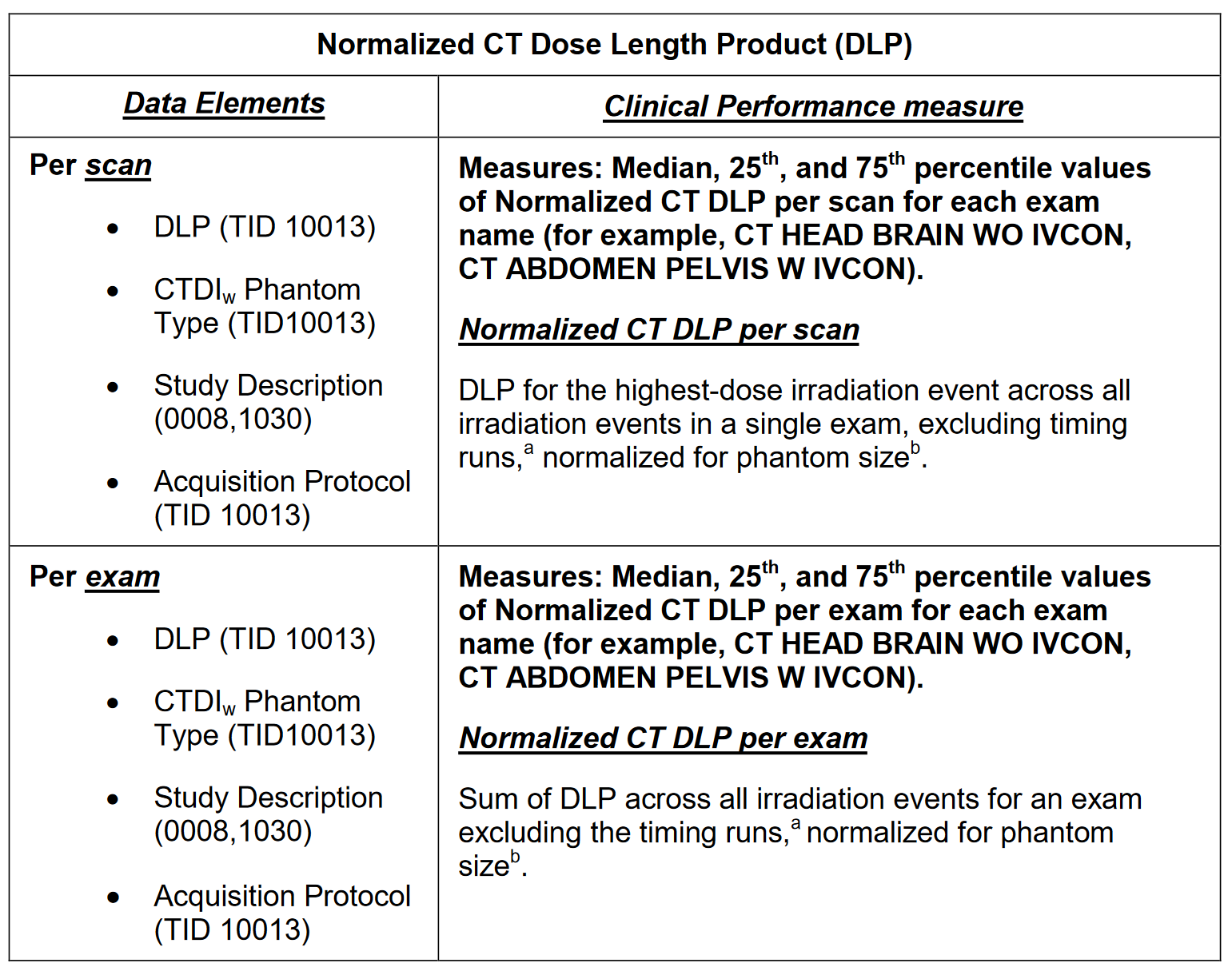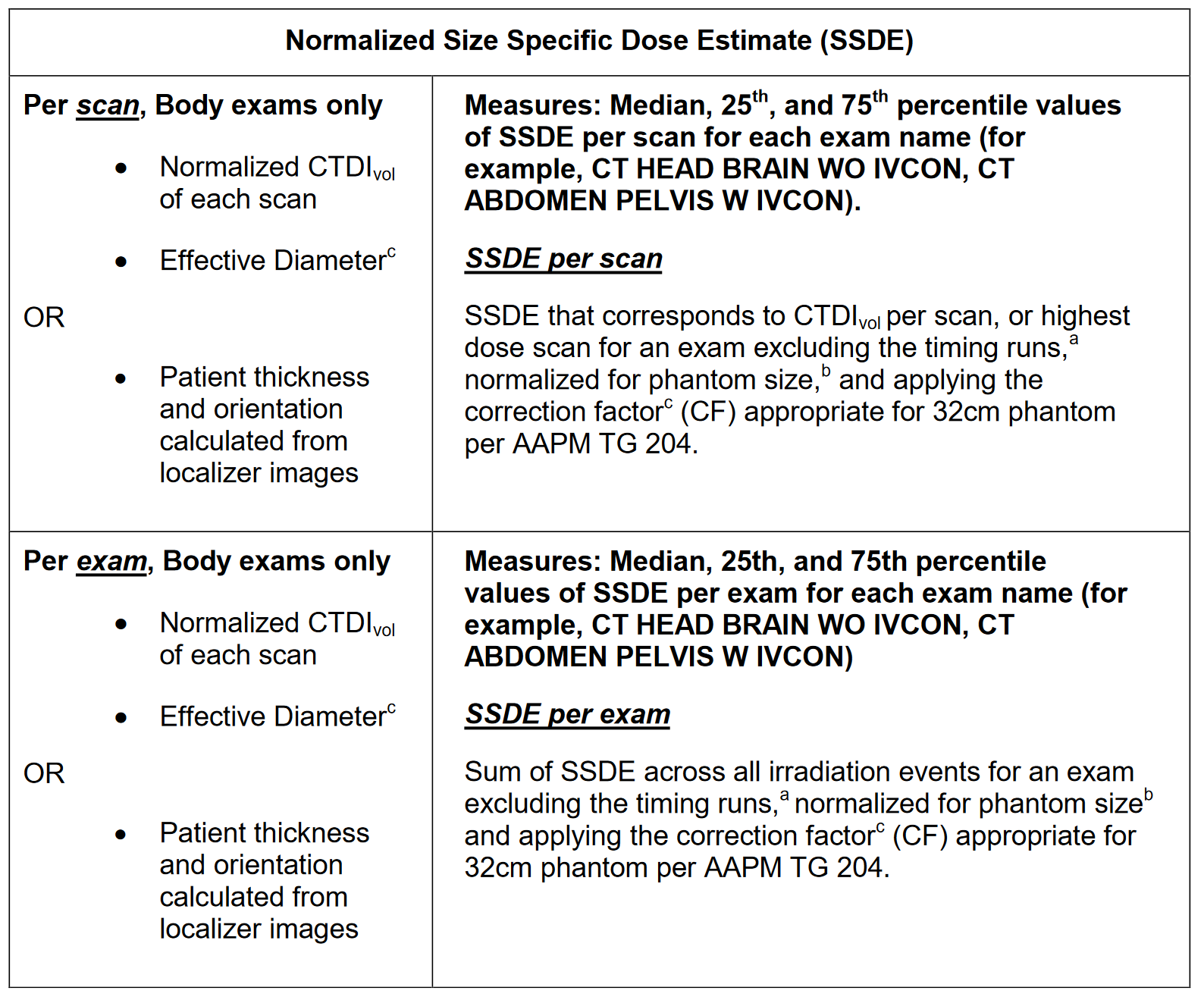# DIR Measures

###### Dose indices for head exams are normalized to a 16cm phantom, and dose indices for body exams are normalized to a 32cm phantom for comparison. For exams involving head region, using a phantom size 32 the normalized CTDIvol is obtained by multiplying the mean CTDIvol and normalized DLP is obtained by multiplying the DLP by the conversion factor of 2.3. For head exams using a 16cm phantom, the normalized CTDIvol and DLP are the same as the original CTDIvol and DLP. For body exams excluding with a phantom size 16, normalized CTDIvol and DLP are calculated by dividing the mean CTDIvol and DLP by a conversion factor of 2.3. For body exams using a 32cm phantom, the normalized CTDIvol and DLP are the same as the original CTDIvol and DLP.From AAPM Report 204: If AP thickness (AP) and Lateral thickness (Lat) both provided, effective diameter (ED) is calculated as ED =a+b*(AP + Lat), where a= -.203128, b= .4958912 If AP is provided but Lat is not provided then ED is calculated as ED = a+b*AP+c*(AP)2 , where a= -3.744858, b= 1.671734, and c=-.01338955 If AP is not provided but Lat is provided then the ED is calculated as ED = a+b*Lat+c*(Lat)2 , where a= 5.899298, b=.3270494, and c =.009978896Correction Factor (CF)= x*exp(-y*ED), where x= 3.704369 and y= .03671937 (CF calculated only for body exams)

Version: March 21, 2014

Did you find it helpful? Yes No

Send feedback